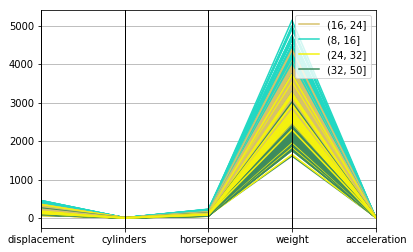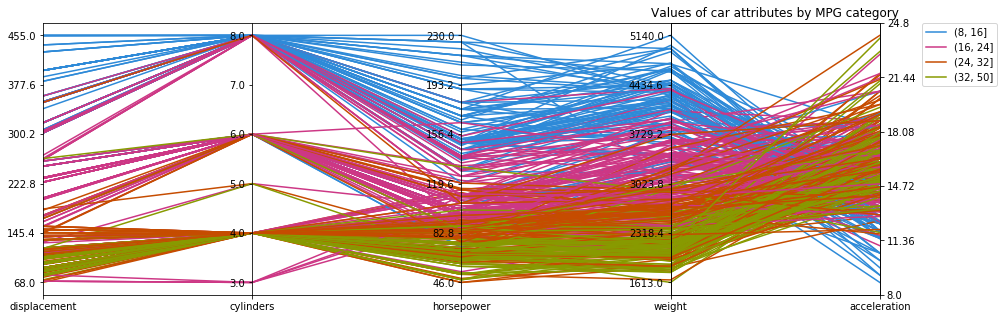# Parallel Coordinates in Matplotlib

In this post we will be exploring the Auto data set from ISLR, which can be found here.

Parellel coordinates is a method for exploring the spread of multidimensional data on a categorical response, and taking a glance at whether there is any trends to the features.

In this post we explore how the various attributes of cars affect MPG.

We start by importing our libraries and data.

In :
import matplotlib.pyplot as plt
%matplotlib inline

import pandas as pd
import numpy as np



MPG is a continuous variable, but to view the effect of of different attributes on MPG in this case we would like it as a categorical variable.

The horsepower feature has some missing values, denoted by the string '?'. As we want to represent this column numerically, we convert the question marks to np.nan.

In :
df['horsepower'] = pd.to_numeric(df['horsepower'].replace('?', np.nan))
df['mpg'] = pd.cut(df['mpg'], [8, 16, 24, 32, 50])


Pandas has a built-in plotting function for creating a parallel coordinates chart using matplotlib.

We can use this by calling it directly with the data frame we’d like to analyse and the target categorical column.

In :
plt.figure()

pd.tools.plotting.parallel_coordinates(
df[['mpg', 'displacement', 'cylinders', 'horsepower', 'weight', 'acceleration']],
'mpg')

plt.show()However we can see clearly that there is some scaling problems with this graph. It is difficult to glean any insight due to the scale of the weight attribute overwhelming the other attributes.

We could just normalise each of the columns, but then we lose information on the values and range.

Instead we will use matplotlib to define each attribute on a single axis that has its own scale.

In :
from matplotlib import ticker

cols = ['displacement', 'cylinders', 'horsepower', 'weight', 'acceleration']
x = [i for i, _ in enumerate(cols)]
colours = ['#2e8ad8', '#cd3785', '#c64c00', '#889a00']

# create dict of categories: colours
colours = {df['mpg'].cat.categories[i]: colours[i] for i, _ in enumerate(df['mpg'].cat.categories)}

# Create (X-1) sublots along x axis
fig, axes = plt.subplots(1, len(x)-1, sharey=False, figsize=(15,5))

# Get min, max and range for each column
# Normalize the data for each column
min_max_range = {}
for col in cols:
min_max_range[col] = [df[col].min(), df[col].max(), np.ptp(df[col])]
df[col] = np.true_divide(df[col] - df[col].min(), np.ptp(df[col]))

# Plot each row
for i, ax in enumerate(axes):
for idx in df.index:
mpg_category = df.loc[idx, 'mpg']
ax.plot(x, df.loc[idx, cols], colours[mpg_category])
ax.set_xlim([x[i], x[i+1]])

# Set the tick positions and labels on y axis for each plot
# Tick positions based on normalised data
# Tick labels are based on original data
def set_ticks_for_axis(dim, ax, ticks):
min_val, max_val, val_range = min_max_range[cols[dim]]
step = val_range / float(ticks-1)
tick_labels = [round(min_val + step * i, 2) for i in range(ticks)]
norm_min = df[cols[dim]].min()
norm_range = np.ptp(df[cols[dim]])
norm_step = norm_range / float(ticks-1)
ticks = [round(norm_min + norm_step * i, 2) for i in range(ticks)]
ax.yaxis.set_ticks(ticks)
ax.set_yticklabels(tick_labels)

for dim, ax in enumerate(axes):
ax.xaxis.set_major_locator(ticker.FixedLocator([dim]))
set_ticks_for_axis(dim, ax, ticks=6)
ax.set_xticklabels([cols[dim]])

# Move the final axis' ticks to the right-hand side
ax = plt.twinx(axes[-1])
dim = len(axes)
ax.xaxis.set_major_locator(ticker.FixedLocator([x[-2], x[-1]]))
set_ticks_for_axis(dim, ax, ticks=6)
ax.set_xticklabels([cols[-2], cols[-1]])

# Remove space between subplots

plt.legend(
[plt.Line2D((0,1),(0,0), color=colours[cat]) for cat in df['mpg'].cat.categories],
df['mpg'].cat.categories,